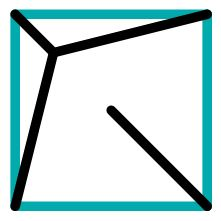BrainDen.com - Brain Teasers
• 1

# Shortest set of lines in a square, revisited

## Question

Recently we considered the shortest roadway that connects the four corners of a square.

Here we seek the shortest set of line segments, one attached to each of a square's corners, that need not connect with each other. Instead, what we ask of the line segments is that is they will block any ray of light attempting to pass through the square.

## Recommended Posts

• 1

Optimizing Captain Ed’s answer a little more

Spoiler

Instead of using a right angle with the North side and West side, consider rotating that so it’s oriented like a V (with a right angle at the bottom). Now instead of having those two lines meet at the northwest corner, push the intersection toward the center of the square a bit and draw a line from the northwest corner to the intersection, so instead of a V it looks like a Y.

To make the math easier, I’ll re-scale distances so the distance from the center of the square to a corner is now defined as 1. If you move the intersection toward the center of the square by a distance of X, and you think of the original North side and West side as hypotenuses of a right triangle with the other two sides of the right triangle having length 1, then the new length for each of the hypotenuses will be
sqrt(A2 + B2)
sqrt(1 + [1-X]2)
sqrt(1 + 1 – 2X + X2)
sqrt(X2 – 2X + 2)
The total length of the lines (excluding the diagonal line from the southeast corner to the center) is twice that length (to count both the North and West lines) plus X.
X + 2 sqrt(X2 – 2X + 2)
Take the derivative of that with respect to X to find out where it reaches a minimum, doing a substitution so you can calculate the derivative
u =  X2 – 2X + 2
du/dx = 2X – 2
d/dx = 1 + d/du [2 sqrt(u)] du/dx
= 1 + (u-1/2) (2X – 2)
= 1 + (2X – 2) / sqrt(X2 – 2X + 2)
The derivative is zero at
2X – 2 = sqrt(X2 – 2X + 2)
4X2 – 8X + 4 = X2 – 2X + 2
3X2 – 6X + 2 = 0
X = [6 ± sqrt(36 – 24)] / 6 = 1 ± sqrt(12)/6
Since X being greater than 1 makes no sense, just consider the negative term
X = 1 – sqrt(12)/6 = 1 – sqrt(1/3) ~= 0.4226

So move the North and West lines so they don’t intersect at the northwest corner and instead intersect ~0.5774 units away from the square’s center (remember that a unit is now defined as the distance from the square’s center to a corner) and draw a line the rest of the way from that intersection to the northwest corner. The total length (again excluding the diagonal line from the southeast corner) would be
0.4226 + 2 sqrt(12 + [sqrt(1/3)]2) ~= 2.732
compared to using the edges of the square which would have length
2 sqrt(12 + 12) ~= 2.828

Adding back the length of the diagonal from the southeast corner to the center of the square (which is just 1 the way I’ve defined distances) and converting from the units I’m using to units where the length of an edge of the square is 1 gives the total length at
(2.732 + 1) / sqrt(2) ~= 2.639

And it looks (approximately) like this##### Share on other sites

• 0

Oh well, here’s an upper bound, as it’s an easy answer:

draw from the center to each corner. So for a unit square, total length 2 sqrt(2).

Im not proud of it, but it’s a starting point

## Join the conversation

You can post now and register later. If you have an account, sign in now to post with your account.×   Pasted as rich text.   Paste as plain text instead

Only 75 emoji are allowed.

×   Your previous content has been restored.   Clear editor

×   You cannot paste images directly. Upload or insert images from URL.